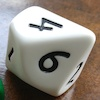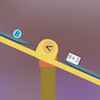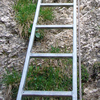# Resources tagged with: Mathematical reasoning & proof

Filter by: Content type:
Age range:
Challenge level:

### There are 96 results

Broad Topics > Thinking Mathematically > Mathematical reasoning & proof### Aba

##### Age 11 to 14Challenge Level

In the following sum the letters A, B, C, D, E and F stand for six distinct digits. Find all the ways of replacing the letters with digits so that the arithmetic is correct.### Eleven

##### Age 11 to 14Challenge Level

Replace each letter with a digit to make this addition correct.### More Mathematical Mysteries

##### Age 11 to 14Challenge Level

Write down a three-digit number Change the order of the digits to get a different number Find the difference between the two three digit numbers Follow the rest of the instructions then try. . . .### Chocolate Maths

##### Age 11 to 14Challenge Level

Pick the number of times a week that you eat chocolate. This number must be more than one but less than ten. Multiply this number by 2. Add 5 (for Sunday). Multiply by 50... Can you explain why it. . . .### Is it Magic or Is it Maths?

##### Age 11 to 14Challenge Level

Here are three 'tricks' to amaze your friends. But the really clever trick is explaining to them why these 'tricks' are maths not magic. Like all good magicians, you should practice by trying. . . .### Gabriel's Problem

##### Age 11 to 14Challenge Level

Gabriel multiplied together some numbers and then erased them. Can you figure out where each number was?### Tis Unique

##### Age 11 to 14Challenge Level

This addition sum uses all ten digits 0, 1, 2...9 exactly once. Find the sum and show that the one you give is the only possibility.### Top-heavy Pyramids

##### Age 11 to 14Challenge Level

Use the numbers in the box below to make the base of a top-heavy pyramid whose top number is 200.##### Age 11 to 14Challenge Level

Make a set of numbers that use all the digits from 1 to 9, once and once only. Add them up. The result is divisible by 9. Add each of the digits in the new number. What is their sum? Now try some. . . .##### Age 5 to 11Challenge Level

Who said that adding couldn't be fun?### The Genie in the Jar

##### Age 11 to 14Challenge Level

This jar used to hold perfumed oil. It contained enough oil to fill granid silver bottles. Each bottle held enough to fill ozvik golden goblets and each goblet held enough to fill vaswik crystal. . . .### Largest Product

##### Age 11 to 14Challenge Level

Which set of numbers that add to 10 have the largest product?### Calendar Capers

##### Age 11 to 14Challenge Level

Choose any three by three square of dates on a calendar page...### Sticky Numbers

##### Age 11 to 14Challenge Level

Can you arrange the numbers 1 to 17 in a row so that each adjacent pair adds up to a square number?### Even So

##### Age 11 to 14Challenge Level

Find some triples of whole numbers a, b and c such that a^2 + b^2 + c^2 is a multiple of 4. Is it necessarily the case that a, b and c must all be even? If so, can you explain why?### One O Five

##### Age 11 to 14Challenge Level

You can work out the number someone else is thinking of as follows. Ask a friend to think of any natural number less than 100. Then ask them to tell you the remainders when this number is divided by. . . .### Square Subtraction

##### Age 7 to 11Challenge Level

Look at what happens when you take a number, square it and subtract your answer. What kind of number do you get? Can you prove it?### What Numbers Can We Make Now?

##### Age 11 to 14Challenge Level

Imagine we have four bags containing numbers from a sequence. What numbers can we make now?### Marbles

##### Age 11 to 14Challenge Level

I start with a red, a green and a blue marble. I can trade any of my marbles for two others, one of each colour. Can I end up with five more blue marbles than red after a number of such trades?### Always, Sometimes or Never?

##### Age 5 to 11Challenge Level

Are these statements relating to odd and even numbers always true, sometimes true or never true?### Always the Same

##### Age 11 to 14Challenge Level

Arrange the numbers 1 to 16 into a 4 by 4 array. Choose a number. Cross out the numbers on the same row and column. Repeat this process. Add up you four numbers. Why do they always add up to 34?### More Marbles

##### Age 11 to 14Challenge Level

I start with a red, a blue, a green and a yellow marble. I can trade any of my marbles for three others, one of each colour. Can I end up with exactly two marbles of each colour?##### Age 11 to 14Challenge Level

A little bit of algebra explains this 'magic'. Ask a friend to pick 3 consecutive numbers and to tell you a multiple of 3. Then ask them to add the four numbers and multiply by 67, and to tell you. . . .### 9 Weights

##### Age 11 to 14Challenge Level

You have been given nine weights, one of which is slightly heavier than the rest. Can you work out which weight is heavier in just two weighings of the balance?### Less Is More

##### Age 5 to 11Challenge Level

Use your knowledge of place value to try to win this game. How will you maximise your score?### More Number Pyramids

##### Age 11 to 14Challenge Level

When number pyramids have a sequence on the bottom layer, some interesting patterns emerge...### Online

##### Age 7 to 11Challenge Level

A game for 2 players that can be played online. Players take it in turns to select a word from the 9 words given. The aim is to select all the occurrences of the same letter.### Go Forth and Generalise

##### Age 11 to 14

Spotting patterns can be an important first step - explaining why it is appropriate to generalise is the next step, and often the most interesting and important.### Tourism

##### Age 11 to 14Challenge Level

If you can copy a network without lifting your pen off the paper and without drawing any line twice, then it is traversable. Decide which of these diagrams are traversable.### Seven Squares - Group-worthy Task

##### Age 11 to 14Challenge Level

Choose a couple of the sequences. Try to picture how to make the next, and the next, and the next... Can you describe your reasoning?### Making Pathways

##### Age 7 to 11Challenge Level

Can you find different ways of creating paths using these paving slabs?### Always, Sometimes or Never? Number

##### Age 7 to 11Challenge Level

Are these statements always true, sometimes true or never true?### Cows and Sheep

##### Age 7 to 11Challenge Level

Use your logical reasoning to work out how many cows and how many sheep there are in each field.### Interactive Balance

##### Age 5 to 11Challenge Level

In this simulation of a balance, you can drag numbers and parts of number sentences on to the trays. Have a play!### Reasoning: Identifying Opportunities (article)

##### Age 5 to 11

In this article for primary teachers we consider in depth when we might reason which helps us understand what reasoning 'looks like'.### Elevenses

##### Age 11 to 14Challenge Level

How many pairs of numbers can you find that add up to a multiple of 11? Do you notice anything interesting about your results?##### Age 11 to 14Challenge Level

Powers of numbers behave in surprising ways. Take a look at some of these and try to explain why they are true.### What Numbers Can We Make?

##### Age 11 to 14Challenge Level

Imagine we have four bags containing a large number of 1s, 4s, 7s and 10s. What numbers can we make?### The Triangle Game

##### Age 11 to 16Challenge Level

Can you discover whether this is a fair game?### Take One Example

##### Age 5 to 11

This article introduces the idea of generic proof for younger children and illustrates how one example can offer a proof of a general result through unpacking its underlying structure.### Reasoning: the Journey from Novice to Expert (article)

##### Age 5 to 11

This article for primary teachers suggests ways in which we can help learners move from being novice reasoners to expert reasoners.### Children at Large

##### Age 11 to 14Challenge Level

There are four children in a family, two girls, Kate and Sally, and two boys, Tom and Ben. How old are the children?##### Age 7 to 14Challenge Level

I added together some of my neighbours' house numbers. Can you explain the patterns I noticed?### Dicing with Numbers

##### Age 11 to 14Challenge Level

In how many ways can you arrange three dice side by side on a surface so that the sum of the numbers on each of the four faces (top, bottom, front and back) is equal?### Tri-colour

##### Age 11 to 14Challenge Level

Six points are arranged in space so that no three are collinear. How many line segments can be formed by joining the points in pairs?### 1 Step 2 Step

##### Age 11 to 14Challenge Level

Liam's house has a staircase with 12 steps. He can go down the steps one at a time or two at time. In how many different ways can Liam go down the 12 steps?### Disappearing Square

##### Age 11 to 14Challenge Level

Do you know how to find the area of a triangle? You can count the squares. What happens if we turn the triangle on end? Press the button and see. Try counting the number of units in the triangle now. . . .### Take Three from Five

##### Age 11 to 16Challenge Level

Caroline and James pick sets of five numbers. Charlie chooses three of them that add together to make a multiple of three. Can they stop him?### Greetings

##### Age 11 to 14Challenge Level

From a group of any 4 students in a class of 30, each has exchanged Christmas cards with the other three. Show that some students have exchanged cards with all the other students in the class. How. . . .### What Do You Need?

##### Age 7 to 11Challenge Level

Four of these clues are needed to find the chosen number on this grid and four are true but do nothing to help in finding the number. Can you sort out the clues and find the number?